• No products in the cart.

# Ncert Solutions for Class 10 Maths

## NCERT SOLUTIONS FOR CLASS 10 MATHS

ToppersCBSE is providing NCERT solutions for CBSE and various state boards on India. Math is always considered as toughest subject to understand. Most of students faces much difficulties to understand math. So we have prepared NCERT solutions for class 10 Math as per marking schemes and with very easy language. Exams are prepared as per the NCERT guidelines. We have used simplest methods to solve the numerical.

These Class 10 maths Ncert solutions are the key for success in board exams for math subject. So it is very important to go through NCERT solutions for each and every student. These are very important if you want to excel in school and board exams. From class 6th to 10th, questions in ncert books are prepared after a great study. You will find that every question has involved a deep concept. In the same way ncert solutions for class 10 maths also help us to understand the core concepts of class 10 math. But students must select these solutions very wisely. Toppers CBSE has done a tremendous job to prepare ncert solutions for class 10 maths and other subjects.

### NCERT SOLUTIONS OF CLASS  10 MATHS- FEATURES

• Simplest methods are used to solve the numerical.
• Best way of writing of solutions are given in our NCERT solutions of math.
• Covered each concepts and numericals.
• Our NCERT Solutions are very helpful for quick revisions and recaps of whole syllabus.
• NCERT Solutions class 10 maths are in very attractive format so these will help the students to create interest in maths.
• Math class 10 is scoring subject so our solutions will help to score good marks in exams.

## NCERT Solutions for Class 10 Maths- Chapterwise

### Here see Some Demo of our best

#### NCERT Solutions for CLASS 10 Maths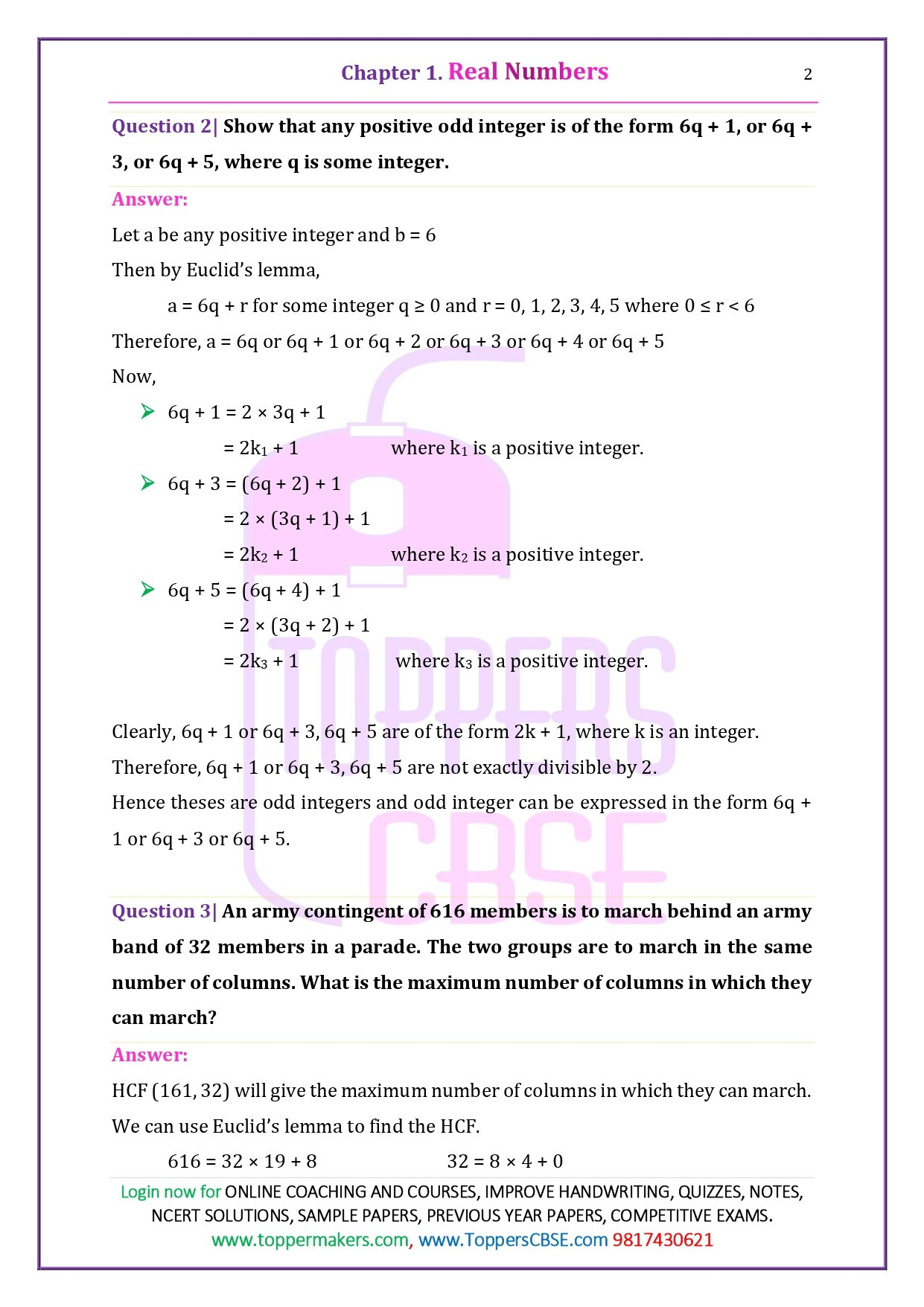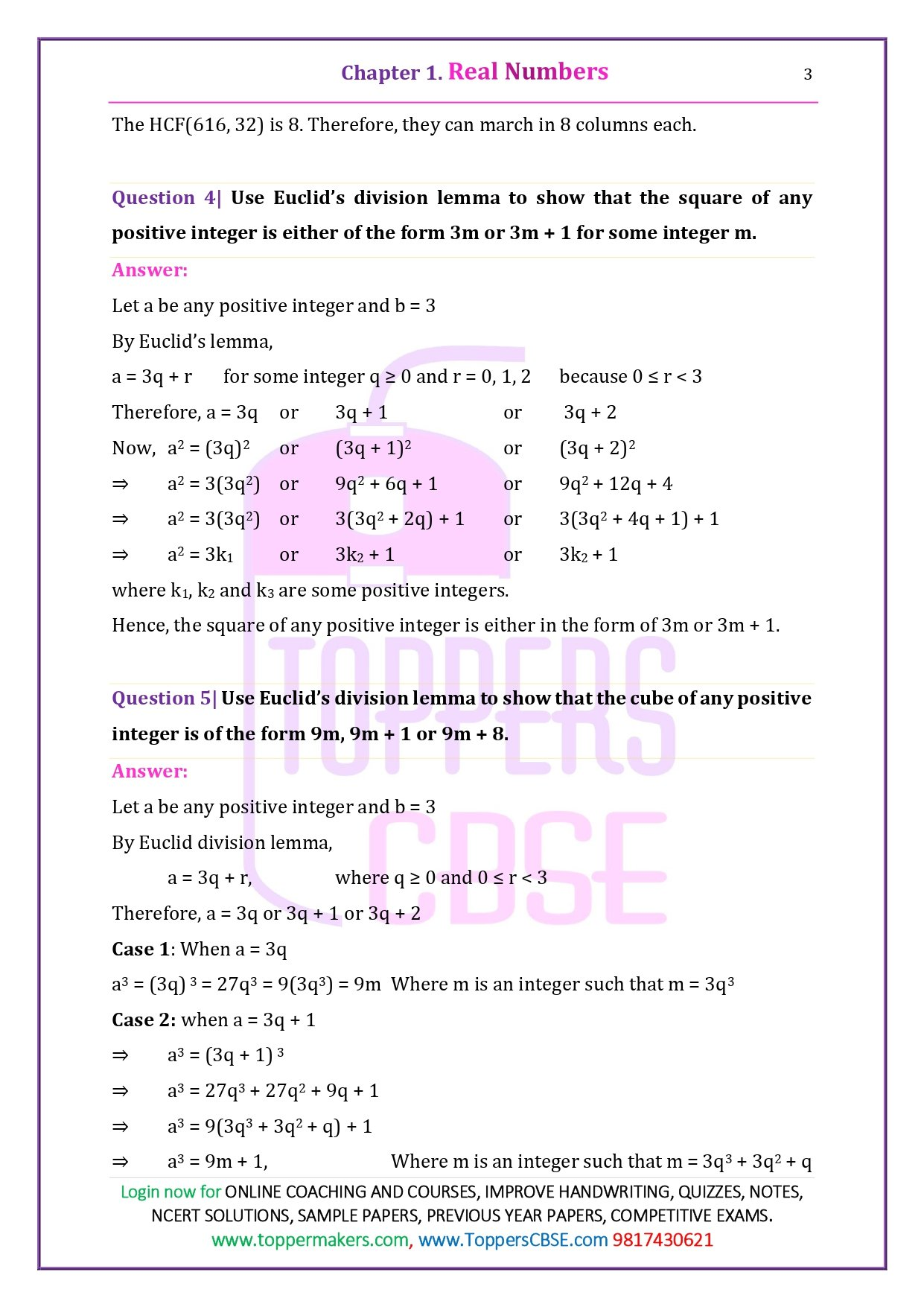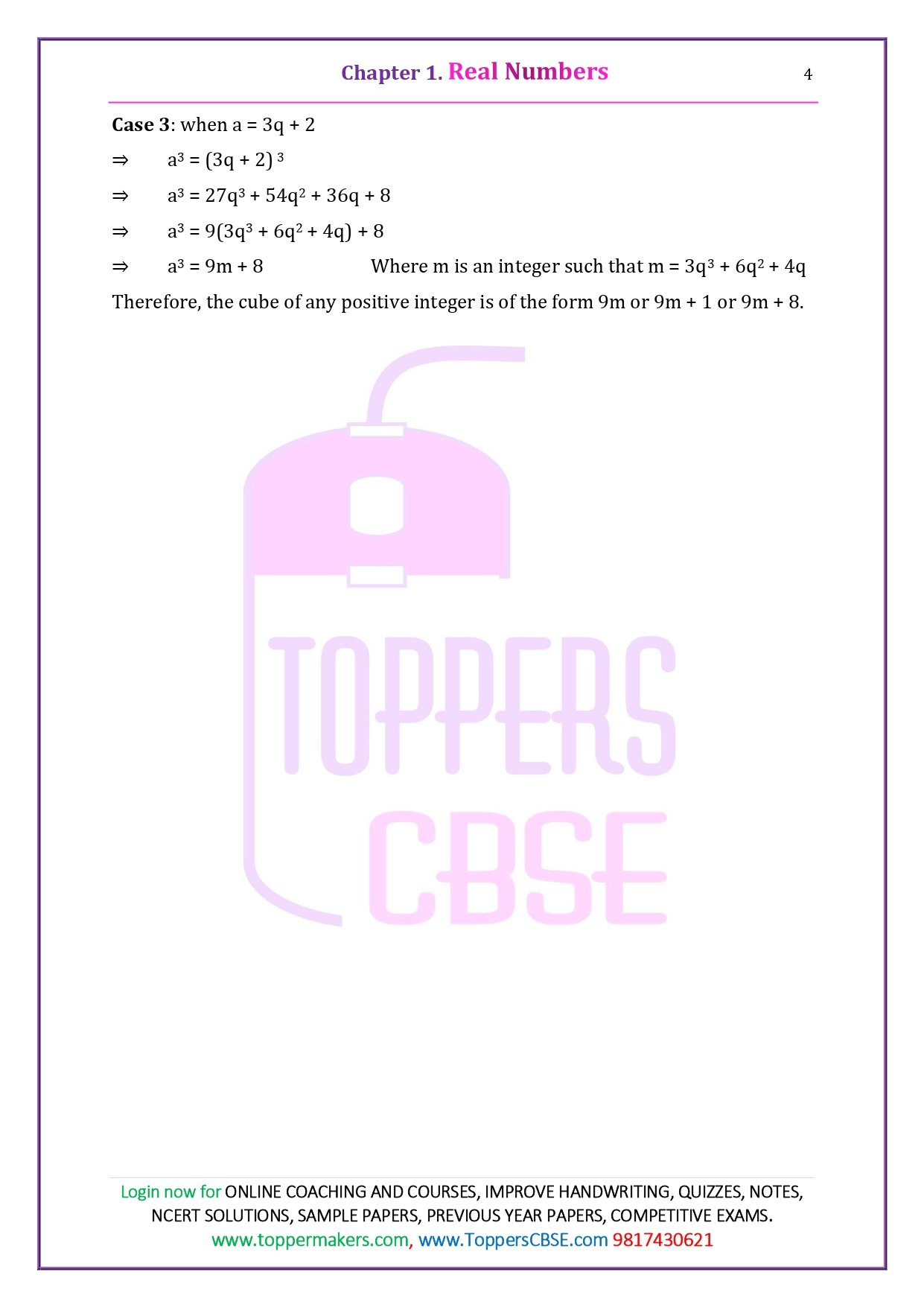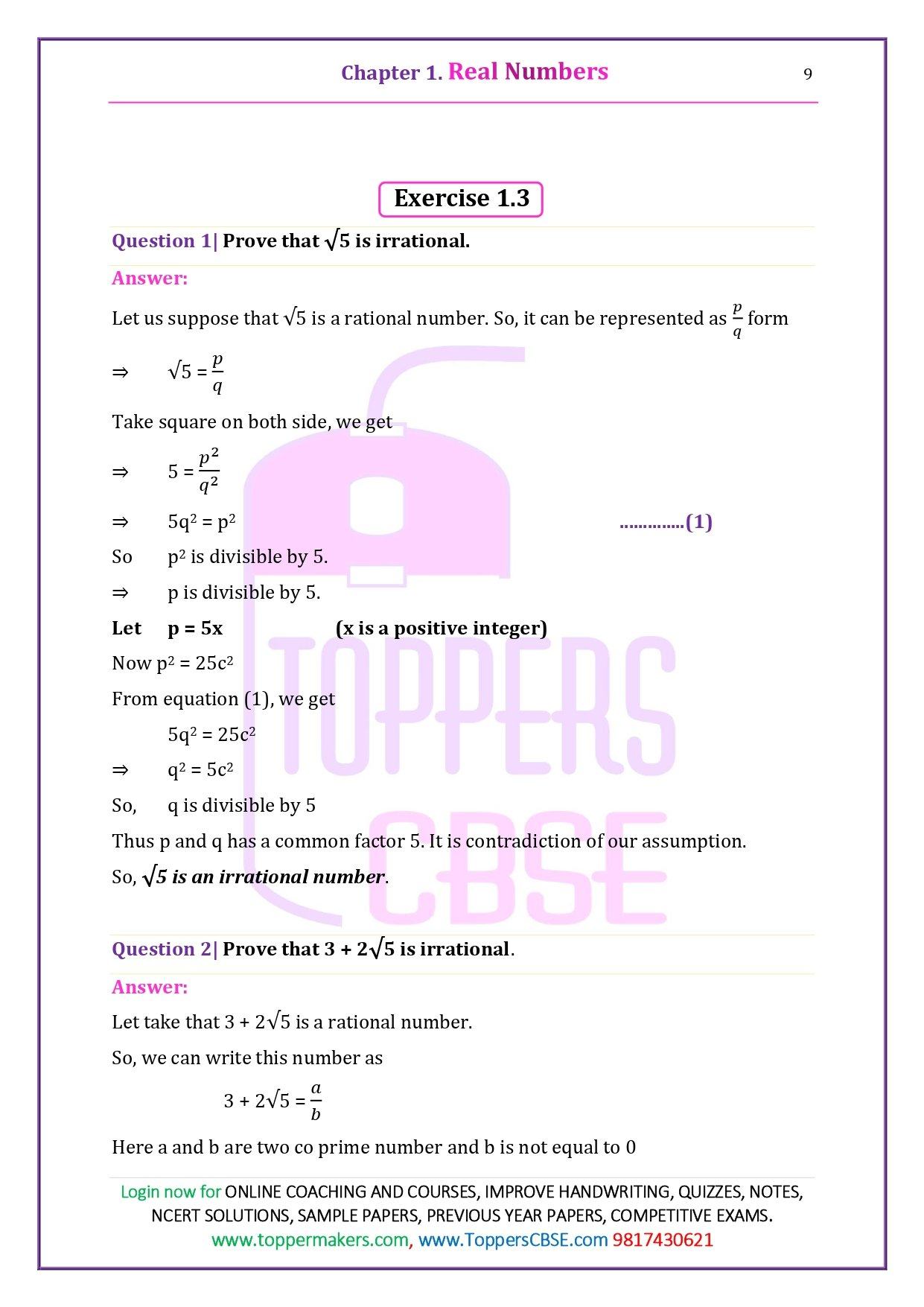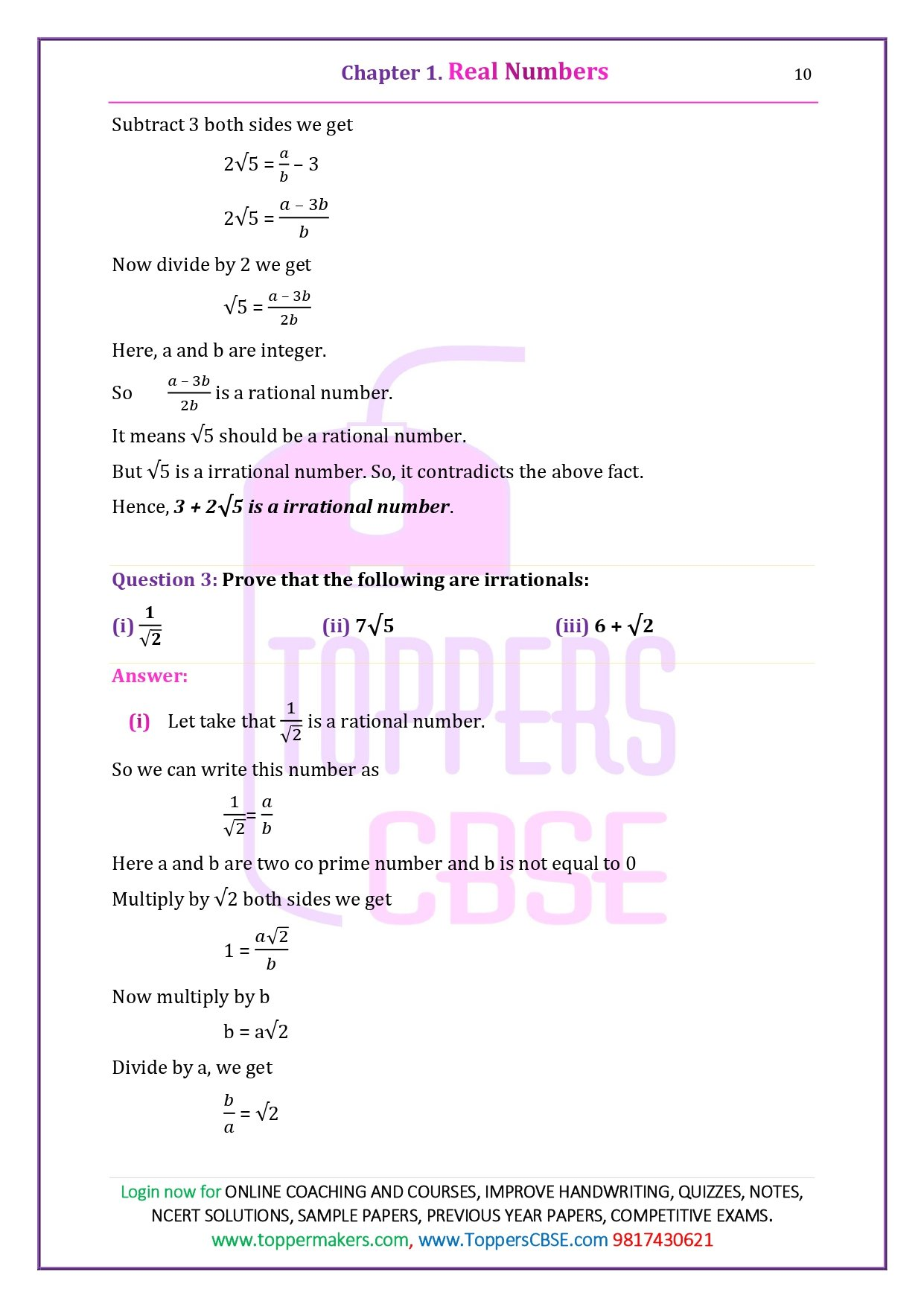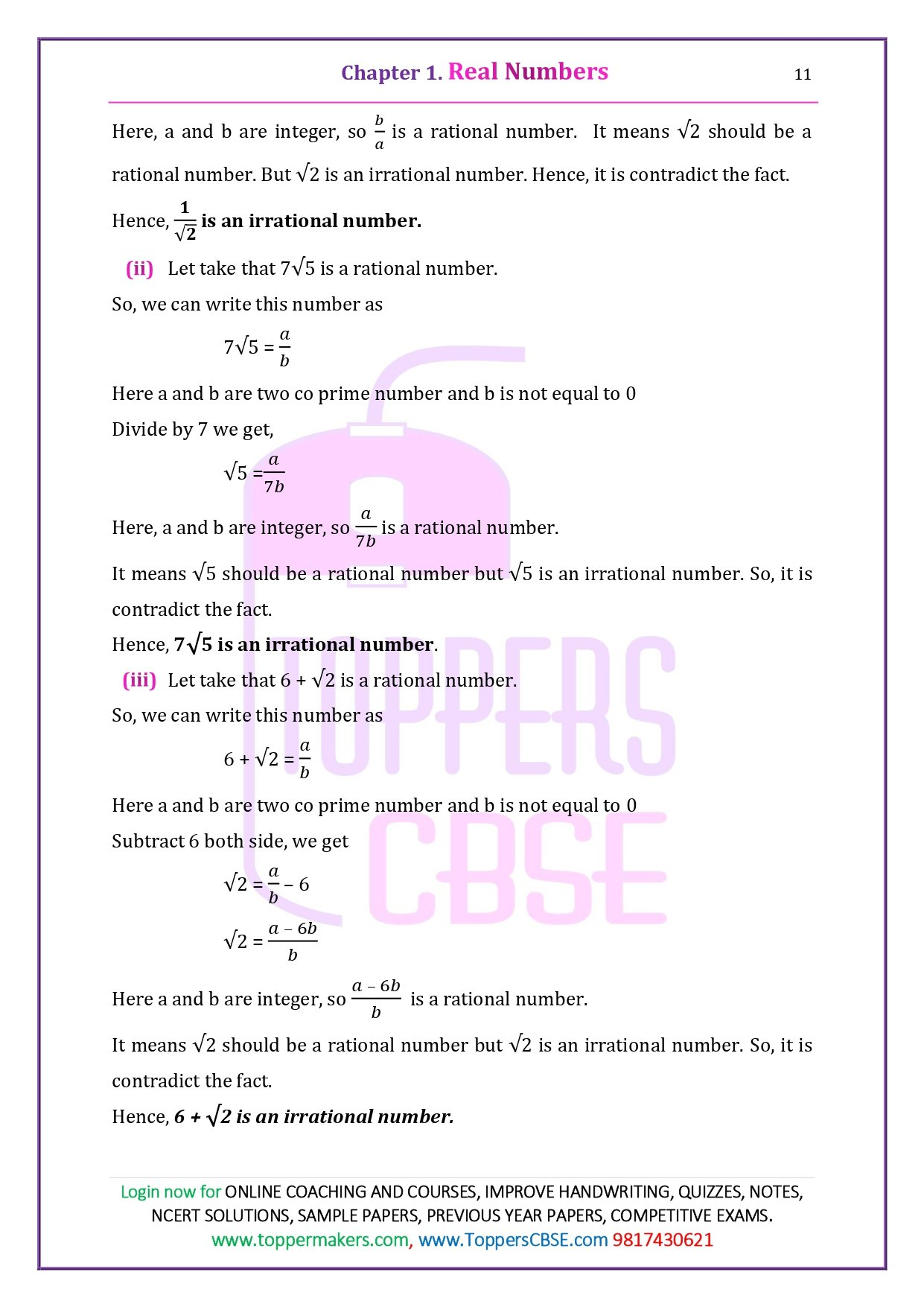### Chapter 1 Real Numbers

In class 10 chapter 1 , students will explore real and irrational numbers. The chapter begins with Euclid’s division lemma, which states that “given positive integers a and b, there exist unique integers qar satisfying a = bq + r, 0≤r<b”. The Euclidean division algorithm is based on this lemma and is used to compute the HCF of two given positive integers. Therefore, the Fundamental Theorem of Arithmetic is defined, which is used to find the LCM and HCF of two positive integers. Then the concept of irrational number, rational number and decimal expansion of rational numbers is explained using the theorem.

Topics Covered in this chapter are- Fundamental Theorem of Arithmetic – statements after reviewing work done before and after illustrating and motivating through examples, Proofs of irrationality of √2, √3, √5.

 NCERT Solutions for Class 10 Maths Chapter 1 Real Numbers Exercises Exercise 1.1 Exercise 1.2 Exercise 1.3 Exercise 1.4

### Chapter 2 Polynomials

Concept Covered: Defining the degree of a polynomial, a linear polynomial, a quadratic polynomial, and a cubic polynomial.

#### Exercise 2.1 contains

• Finding the number of zeros in a graph
• The geometric meaning of the zeros of a polynomial.

#### Exercise 2.2 contains

• Relationship between Zeros and Coefficients of a Polynomial,
• Find the zeros of a quadratic polynomial.

#### Exercise 2.3 contains

• Division algorithm and related questions.

#### Exercise 2.4 contains

• Questions from all concepts of chapter 2.
 NCERT Solutions for Class 10 Maths Chapter 2 Polynomials Exercise 2.1 Exercise 2.2 Exercise 2.3 Exercise 2.4

### Chapter 3 Pair of Linear Equations in Two Variables

#### Exercise 3.1 describes

• How to represent the situation algebraically and graphically.

#### Exercise 3.2 explains

• Methods for solving a pair of linear equations using the graphical method.

#### Exercises 3.3, 3.4, 3.5, and 3.6 explains

• The algebraic method, the elimination method, the cross-multiplication method, and the substitution method.

#### Exercise 3.7 is optional

• Exercise that contains all types of questions. Students must practice these exercises to master the method of solving linear equations.

We will learn- Pair of linear equations in two variables and graphical method of their solution, consistency/ inconsistency. Algebraic conditions for number of solutions. Pair of linear equations in two variables solution – by substitution, by elimination. Simple situational problems.

 NCERT Solutions for Class 10 Maths Chapter 3 Pair of Linear Equations in Two Variables Exercise 3.1 Exercise 3.2 Exercise 3.3 Exercise 3.4 Exercise 3.5 Exercise 3.6 Exercise 3.7

### Other Best Study Materials Class 10 Maths Chapter 3 Pair of Linear Equations in Two Variables at Toppers CBSE

In this chapter, students will discuss the standard form of writing a Quadratic Equation. The chapter properly explain the method of solving the quadratic equation through the completing the square method and factorization method. The chapter finish with the topic on finding the nature of roots which means, a quadratic equation ax² + bx + c = 0 has:

• Two distinct real roots, if b² – 4ac > 0
• Two equal roots, if b² – 4ac = 0
• No real roots, if b² – 4ac < 0

In this Chapter We will Learn – Standard form of a quadratic equation ax2 + bx + c = 0, (a ≠ 0). Solutions of quadratic equations (only real roots) by factorization, and by using quadratic formula. Relationship between discriminant and nature of roots.

 NCERT Solutions for Class 10 Maths Chapter 4 Quadratic Equations Exercises Exercise 4.1 Exercise 4.2 Exercise 4.3 Exercise 4.4

### Chapter 5 Arithmetic Progressions

This chapter introduces students to the new topic of Arithmetic Progression or AP. The chapter contains a total of 4 exercises. In Exercise 5.1, students will find questions about representing a situation in AP form, finding the first term and difference of an AP, and determining whether a series is an AP or not. Exercise 5.2 contains questions on finding the nth term of an AP using the following formula:

an = a + (n-1) d

In the next exercise i.e., 5.3, contains the questions on finding the sum of first n terms of an AP. The last exercise includes higher-level questions based on AP to enhance students’ analytical and problem-solving skills.

In this Chapter We will Learn – Arithmetic Progression, Derivation of the nth term and the sum of the first n terms of A.P. and their application in solving daily life problems.

 NCERT Solutions for Class 10 Maths Chapter 5 Arithmetic Progressions Exercises Exercise 5.1 Exercise 5.2 Exercise 5.3 Exercise 5.4

### Chapter 6 Triangles

In Class 10 CBSE Maths chapter 6, students will study figures of the same shape but not necessarily the same size. The Triangles chapter begins with the concept of a similar and congruent figure. It further explains the condition of the similarity of two triangles and theorems related to the similarity of triangles. Then the areas of similar triangles were explained by using the theorem. At the end of this chapter, the Pythagorean theorem and the inversion of the Pythagorean theorem are discussed.

In this Chapter We will Learn – Definitions, examples, counter examples of similar triangles.

1. Prove that if a line is drawn parallel to the one side of a triangle to intersect the other two sides in the distinct points and the other two sides are divided in the same ratio.
2. If a line divides the two sides of a triangle in the same ratio, the line is parallel to the third side.
3. If in two triangles, the corresponding angles are equal, their corresponding sides are proportional and the triangles are similar.
4. If the corresponding sides of two triangles are proportional, their corresponding angles are equal and the two triangles are similar.
5. If one angle of a triangle is equal to one angle of the another triangle and sides including these angles are proportional, and the two triangles are similar.
 NCERT Solutions for Class 10 Maths Chapter 6 Triangles Exercises Exercise 6.1 Exercise 6.2 Exercise 6.3 Exercise 6.4 Exercise 6.5 Exercise 6.6

### Chapter 7 Coordinate Geometry

In the chapter 7, students will learn how to find the distance between two points whose coordinates are given and the area of the triangle formed by the three given points. Students will also learn how to find the coordinates of a point that bisects a line joining the two given points in a given ratio. In the end, students will be introduced to the distance formula, the section formula, and the area of a triangle in this Coordinate Geometry chapter.

In this Chapter We will Learn – the concepts of coordinate geometry, graphs of linear equations. Distance formula. Section formula (internal division).

 NCERT Solutions for Class 10 Maths Chapter 7 Coordinate Geometry Exercises Exercise 7.1 Exercise 7.2 Exercise 7.3 Exercise 7.4

### Chapter 8 Introduction to Trigonometry

This chapter introduces students about trigonometry. They will study about some ratios of a right triangle with respect to its acute angles, called trigonometric ratios of angles. The chapter also defines trigonometric ratios for angles 00 and 900. Furthermore, students will know how to calculate trigonometric ratios for specific angles and establish some identities involving these ratios, called trigonometric identities.

In this Chapter We will Learn – Trigonometric ratios of an acute angle of a right-angled triangle. Proof (well defined); motivate the ratios whichever are defined at 0o and 90o. Values of the trigonometric ratios of 300, 450 and 600. Relationships between the ratios. Trigonometric Identities: Proof and applications of the identity sin2A + cos2A = 1. Only simple identities to be given.

 NCERT Solutions for Class 10 Maths Chapter 8 Introduction to Trigonometry Exercises Exercise 8.1 Exercise 8.2 Exercise 8.3 Exercise 8.4

### Chapter 9 Some Applications of Trigonometry

In this chapter, students will study the applications of trigonometry here. It is used in geography, navigation, map construction, and determining the position of an island in relation to latitudes and longitudes. Students will see how trigonometry is used to find the heights and distances of various objects without actually measuring them. They will become familiar with the topics of the line of sight, angle of elevation, and angle of depression.

In this Chapter We will Learn – Height and Distance: Angle of elevation, and Angle of Depression. Basic problems on heights and distances. Problems should not contain more than two right triangles. Angles of elevation/depression should be only 30°, 45°, or 60°.

 NCERT Solutions for Class 10 Maths Chapter 9 Some Applications of Trigonometry Exercises Exercise 9.1

### Chapter 10 Circles

In earlier classes, students learned about a circle and various terms related to a circle, such as chord, segment, arc, etc. In chapter 10, students will study the various problem that occur when a circle and a line are placed in a plane. So they get thoroughly acquainted with the concept of a tangent to a circle and the number of tangents from the point on a circle.

In this Chapter We will Learn – Tangent to a circle, point of contact

• Prove that the tangent at any point of a circle is perpendicular to the radius through the point of contact.
• Prove that lengths of tangents drawn from an external point to a circle are equal.
 NCERT Solutions for Class 10 Maths Chapter 10 Circles Exercises Exercise 10.1 Exercise 10.2

### Chapter 11 Constructions

This chapter consists of a total of two exercises. Students who have learned about construction in earlier lessons will also help them. In Exercise 11.1, students will discuss how to bisect a line, while in Exercise 11.2, they will also learn the construction of tangents to a circle. The methods and steps for the construction are explained, and some examples are additionally given to make it more clear to the students.

In this Chapter We will Learn – Division of a line segment in a given ratio (internally), Tangent to a circle from the point outside it, and construction of a triangle similar to a given triangle.

 NCERT Solutions for Class 10 Maths Chapter 11 Constructions Exercises Exercise 11.1 Exercise 11.2

### Chapter 12 Areas Related to Circles

This chapter begins with the concepts of circumference and area of a circle. Using this concept, the chapter goes on to explain how to find the area of a sector and a segment of a given circular region. In addition, students will learn to calculate the areas of some combinations of plane figures involving circles or their parts.

In this Chapter We will Learn – Area of sectors and segments of a circle. Problems based on areas and perimeter/circumference. Calculating the area of segment of a circle, problems should be restricted to central angle of 60°, 90° and 120° only.

 NCERT Solutions for Class 10 Maths Chapter 12 Areas Related to Circle Exercises Exercise 12.1 Exercise 12.2 Exercise 12.3

### Chapter 13 Surface Areas and Volumes

There are five exercises in Chapter 13. The first exercise consists of questions based on determining the surface area of an object created by joining any two basic bodies, i.e., a cuboid, a cone, a cylinder, a sphere, and a hemisphere. In the exercise, question 13.2 are based on finding the volume of objects formed by combining any of the cuboid, cone, cylinder, sphere, and hemisphere. Exercise 13.3 deals with questions in which a body is transformed from one shape to another. Exercise 13.4 is based on determining the volume, curved surface, and total surface of a frustum of a cone. The final exercise is optional and contains high-level questions based on all the topics in the chapter.

In this Chapter We will Learn – Surface areas and volumes of combinations of any two of the following: cubes, cuboids, spheres, hemispheres, and right circular cylinders/cones.

 NCERT Solutions for Class 10 Maths Chapter 13 Surface Areas and Volumes Exercises Exercise 13.1 Exercise 13.2 Exercise 13.3 Exercise 13.4 Exercise 13.5

### Chapter 14 Statistics

Here, students will discuss the numerical representation of ungrouped data into grouped data and find the mean, mode, and median. The concept of cumulative frequency, cumulative frequency distribution, and how to draw cumulative frequency curves will also be explained.

In this Chapter We will Learn – Mean, median, and mode of grouped data (bimodal situation to be avoided).

 NCERT Solutions for Class 10 Maths Chapter 14 Statistics Exercises Exercise 14.1 Exercise 14.2 Exercise 14.3 Exercise 14.4

### Chapter 15 Probability

The final chapter deals with Probability. The chapter begins with a theoretical approach to probability. Subsequently, the chapter explains the difference between experimental probability and theoretical probability. Various examples are given for effective explanation. So, before going through the practice problems, students must first solve the CBSE Maths examples.

In this Chapter We will Learn – Classical definition of probability. and simple problems on the probability of an event.

 NCERT Solutions for Class 10 Maths Chapter 15 Probability Exercises Exercise 15.1 Exercise 15.2

## How NCERT Solutions for Class 10 Maths help you in the New Pattern of CBSE Board

Recently CBSE announced its new pattern of board exam for class 10. Now onwards students will appear for two exams in their class 10. Maths is the most scoring subject for all students and it needs practice of questions and understanding the applications of formulas and concepts in the numerical.

Exercise of NCERT is most important and NCERT solutions for class 10 Maths prepared by experts of Toppers CBSE can help you a lot. According to the new pattern of CBSE board Maths, the subject consists of the following weightage.

 Unit Title Marks Weightage 1 Number System 06 2 Algebra 20 3 Coordinate Geometry 06 4 Geometry 15 5 Trigonometry 12 6 Mensuration 10 7 Statistics And Probability 11 Total Marks 80

## Why are NCERT Solutions for Class 10 Maths important?

The entire study guide was created after a thorough analysis of the subject. According to the most recent NCERT council guidelines, students can locate the NCERT solutions for math class 10 problems. Because many kids have a lot of trouble solving common math problems. They can resolve typical examples under the supervision of teachers using study materials.

1. Class 10 math also includes challenging theories and numerical problems that require extra care. The Toppers CBSE team has also made an effort to give students unique notes on subjects that require more attention.
2. The most frequently asked questions from prior years are thoroughly explained to the pupils. For pupils, it might occasionally be challenging to solve us.
3. A detailed explanation of how to solve ordinary sums is included in each question’s explanation. Our team additionally includes additional idea notes in the study materials.
4. The squad is made up of professionals who have already aced national-level tests. Expert advice given in the form of notes is quite beneficial. Questions and study materials can be found with a methodical approach for students. Any student with a problem can speak with one of our faculty members personally

## How can I efficiently study NCERT Solutions for Class 10 Math?

• A unidirectional approach is important due to the variety of topics covered in the math curriculum.
• With the help of the professionals at Toppers CBSE, students may simply prepare for class 10 math.
• The study material is extremely condensed and efficient, and it may be useful for revision. The team has conducted extensive, in-depth examinations of a variety of questions from prior years in order to maximise benefit to students.
• The preparation of these study resources makes it possible for friends to use them together.

## Why is NCERT Solutions for Class 10 Maths the best?

• Students can review the exercise’s questions well in advance if they want to get top scores on their test. It is accessible to you in PDF format.
• Multiple devices can access this solution. The study materials’ own ideology is developing the fundamental concepts.

## Chapter-wise NCERT Mathematical Solutions for Class 10

Do not be concerned about your math challenges; I can guarantee you that mine are greater. Albrecht Einstein Making simple things complicated is not the goal of mathematics; rather, it is to simplify complicated things. Beginning with class 10, a student’s most crucial year begins. Your subject’s interest and basis were established in this year. Class 10 Math is the most significant subject in terms of academic difficulty.

The academic team at Toppers CBSE has uploaded the NCERT solutions for class 10 math question answers on this page, along with another theoretical requirement for the subject. We have also uploaded all chapter-by-chapter explanations of the theory and questions for class 10 that include objective questions, subjective questions, and solved questions. These resources are very helpful for your board exam as well as entrance exam preparation, including NTSE, a foundation for JEE and NEET.

Q1. How do I get better marks in math in class 10?

Ans. One must adhere closely to the NCERT text book in order to receive good marks in math for class ten. Read the textbook theory and make an effort to develop your own concepts. When writing your notes from the NCERT class 10 math textbook, be sure to include all of the chapter’s key formulas.

Try to complete all of the numerical tasks in the exercise on your own, using the NCERT class 10 math solutions simply as a guide. Build up your knowledge and understanding of the format from class 10 question papers from past years, and then practise with class 10 math practise tests. Always keep in mind to practise well. The finest strategy for achieving perfect marks in math class 10 is numerical math.

Q2. Which math chapters from class 10 are crucial for the CBSE Board Exam?

Ans. There isn’t a specific chapter that has important questions for class 10 math because all chapters are covered. Prepare a strategy to identify the crucial chapter in math for grade 10 when questions worth five points are asked. Some Applications of Trigonometry, Probability, and Pair of Linear Equations in Two Variables are a few examples of such chapters.

Q3. How can I effectively use the NCERT Solutions for Math Class 10?

NCERT solutions for class 10 mathematics must be used in accordance with the guidelines below, for example.

1. Don’t duplicate any homework answers from the class 10 math NCERT solutions.
2. Attempt to determine the appropriate response to each and every question in the exercise; do not jump to the answer right away.
3. Do not use the NCERT solutions for class 10 mathematics to complete your homework; only use them as a resource.

Q4. How many chapters are there in the NCERT and CBSE class 10 math curricula?

Ans. The NCERT class 10 math textbook has 15 chapters, with two to three exercises per chapter that include problems with long answers, short answers, and multiple-choice questions. Each chapter has between 30 and 45 questions. For more practise, students must solve NCERT example.

Q5. Will the math from NCERT class 10 be enough for the CBSE board exam?

Ans. Yes, the NCERT text book is more than sufficient if you are preparing for the CBSE class 10 board exam. However, if you are preparing for the NTSE or JEE foundation, you will need additional resources, such as Toppers CBSE Class 10 Maths notes for JEE.

Related Chapters

• NCERT Solutions For Class 10 Maths
• NCERT Solutions for class 10 Science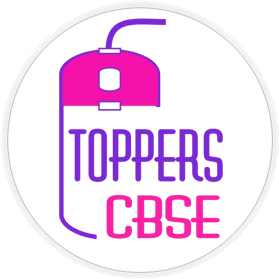Best Online Courses and Live Classes

#### Important Policies

Team members of Toppers CBSE are very proud to announce that we are providing awesomeness to our learners. We think that we are going to help a lot of people to save their better future and life. We hope that we are doing best and will try to improve our resources day by day. CBSE toppers not only meant for toppers in board exams but also meant for creating class toppers. Toppers CBSE is providing Online coaching for CBSE, ICSE, State boards and Competitive exams. Toppers CBSE also contains NCERT solutions, Notes, Sample papers, Quizzes and much more. NCERT solutions for class 6th, 7th, 8th, 9th, 10th. 11th, 12th are vert awesome in pattern and languages. Revision NOTES are prepared by our best team by keeping syllabus and exam pattern in mind. ONLINE COACHING is providing by our Experienced and Dedicated team. Also we are providing online courses for easy learning at any place and any time.

hhhhhhhh